Next: Fundamentals of Quantum Mechanics Up: Collapse of the Wave Previous: Collapse of the Wave

Exercises

1. A He-Ne laser emits radiation of wavelength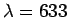nm. How many photons are emitted per second by a laser with a power of 1 mW? What force does such laser exert on a body which completely absorbs its radiation?

2. The ionization energy of a hydrogen atom in its ground state is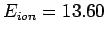eV (1 eV is the energy acquired by an electron accelerated through a potential difference of 1 V). Calculate the frequency, wavelength, and wavenumber of the electromagnetic radiation which will just ionize the atom.

3. The maximum energy of photoelectrons from aluminium is 2.3 eV for radiation of wavelength, and 0.90 eV for radiation of wavelength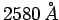. Use this data to calculate Planck's constant, and the work function of aluminium.

4. Show that the de Broglie wavelength of an electron accelerated from rest across a potential differenceis given by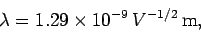whereis measured in volts.

5. If the atoms in a regular crystal are separated by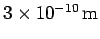demonstrate that an accelerating voltage of about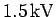would be required to produce an electron diffraction pattern from the crystal.

6. The relationship between wavelength and frequency for electromagnetic waves in a waveguide iswhere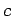is the velocity of light in vacuum. What are the group and phase velocities of such waves as functions ofand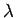?

7. Nuclei, typically of sizem, frequently emit electrons with energies of 1-10 MeV. Use the uncertainty principle to show that electrons of energy 1 MeV could not be contained in the nucleus before the decay.

8. A particle of masshas a wavefunction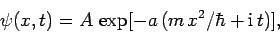whereandare positive real constants. For what potential functiondoes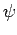satisfy the Schrödinger equation?Next: Fundamentals of Quantum Mechanics Up: Collapse of the Wave Previous: Collapse of the Wave
Richard Fitzpatrick 2010-07-20